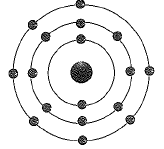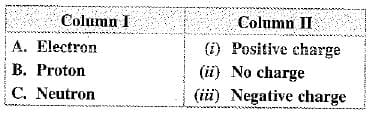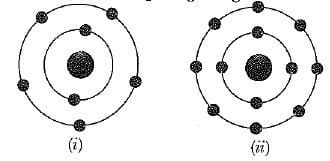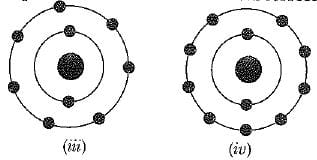UPSC  >  Test: Structure Of The Atom- 2

# Test: Structure Of The Atom- 2 - UPSC

Test Description

## 20 Questions MCQ Test Science & Technology for UPSC CSE - Test: Structure Of The Atom- 2

Test: Structure Of The Atom- 2 for UPSC 2023 is part of Science & Technology for UPSC CSE preparation. The Test: Structure Of The Atom- 2 questions and answers have been prepared according to the UPSC exam syllabus.The Test: Structure Of The Atom- 2 MCQs are made for UPSC 2023 Exam. Find important definitions, questions, notes, meanings, examples, exercises, MCQs and online tests for Test: Structure Of The Atom- 2 below.
Solutions of Test: Structure Of The Atom- 2 questions in English are available as part of our Science & Technology for UPSC CSE for UPSC & Test: Structure Of The Atom- 2 solutions in Hindi for Science & Technology for UPSC CSE course. Download more important topics, notes, lectures and mock test series for UPSC Exam by signing up for free. Attempt Test: Structure Of The Atom- 2 | 20 questions in 20 minutes | Mock test for UPSC preparation | Free important questions MCQ to study Science & Technology for UPSC CSE for UPSC Exam | Download free PDF with solutions
 1 Crore+ students have signed up on EduRev. Have you?
Test: Structure Of The Atom- 2 - Question 1

### Which of the follwing correcctly represent the electronics distribution in the Mg atom ?

Detailed Solution for Test: Structure Of The Atom- 2 - Question 1

To determine the electronic distribution in the Mg (Magnesium) atom, we need to consider its atomic number and the arrangement of electrons in its energy levels.
The atomic number of Mg is 12, which means it has 12 electrons. The electronic distribution can be represented as follows:
- The first energy level (closest to the nucleus) can hold a maximum of 2 electrons.
- The second energy level can hold a maximum of 8 electrons.
- The third energy level can hold a maximum of 8 electrons.
Based on this information, we can determine the correct electronic distribution in the Mg atom:
- The first energy level (1s) will contain 2 electrons.
- The second energy level (2s and 2p) will contain 8 electrons.
- The third energy level (3s) will contain 2 electrons.
Therefore, the correct representation of the electronic distribution in the Mg atom is:
2,8,2
This means that the Mg atom has 2 electrons in the first energy level, 8 electrons in the second energy level, and 2 electrons in the third energy level.
Test: Structure Of The Atom- 2 - Question 2

### Which of the following statement(s) is/are incorrect for anode rays?

Detailed Solution for Test: Structure Of The Atom- 2 - Question 2
Incorrect Statement for Anode Rays:
Statement C: The charge/mass ratio of particles in anode rays is constant.
Explanation:
Anode rays are a stream of positively charged particles that are produced in a discharge tube when a high voltage is applied. These particles are usually positively charged ions or positive atomic or molecular fragments.
The incorrect statement is that the charge/mass ratio of particles in anode rays is constant. This statement is incorrect because the charge/mass ratio of particles in anode rays actually depends on the gas in the discharge tube used to produce them.
Here are the correct statements regarding anode rays:
Statement A: They are deflected by electric and magnetic fields.
- Anode rays are charged particles, so they can be deflected by both electric and magnetic fields.
Statement B: Their charge/mass ratio depends upon the gas in the discharge tube used to produce anode rays.
- The charge/mass ratio of anode rays depends on the nature of the gas in the discharge tube. Different gases produce different charge/mass ratios.
Statement D: They are produced by ionization of gas in the discharge tube.
- Anode rays are produced when gas in the discharge tube is ionized by the high voltage applied to it. The positively charged ions or fragments are then accelerated towards the anode, forming the anode rays.
In conclusion, the incorrect statement is that the charge/mass ratio of particles in anode rays is constant. The charge/mass ratio actually depends on the gas in the discharge tube used to produce anode rays.
Test: Structure Of The Atom- 2 - Question 3

### In the Thomson’s model of atoms, which of the following statements are correct?  (i) The mass of the atoms is assumed to be uniformly distributed over the atom.  (ii) The positive charge is assumed to be uniformly distributed over the atoms.  (iii) The electrons are uniformly distributed in the positively charged sphere.  (iv) The electrons attract each other to stabilise the atom.

Detailed Solution for Test: Structure Of The Atom- 2 - Question 3
Thomson's Model of Atoms
Thomson's model of atoms, also known as the plum pudding model, was proposed by J.J. Thomson in the early 20th century. According to this model, an atom consists of a positively charged sphere in which negatively charged electrons are embedded. Let's analyze each statement from the given options:
(i) The mass of the atoms is assumed to be uniformly distributed over the atom.
- This statement is correct. In Thomson's model, the mass of the atom is assumed to be uniformly distributed throughout the atom.
(ii) The positive charge is assumed to be uniformly distributed over the atoms.
- This statement is incorrect. In Thomson's model, the positive charge is not assumed to be uniformly distributed. Instead, it is concentrated in a sphere.
(iii) The electrons are uniformly distributed in the positively charged sphere.
- This statement is correct. According to Thomson's model, the negatively charged electrons are uniformly distributed in the positively charged sphere.
(iv) The electrons attract each other to stabilize the atom.
- This statement is incorrect. In Thomson's model, there is no mention of electrons attracting each other. Instead, the electrons are embedded in the positively charged sphere, which provides stability to the atom.
Therefore, the correct statements are (i) and (iii). Hence, the answer is (A) (i) and (iii).
Test: Structure Of The Atom- 2 - Question 4

1 mole of diatomic element X2 contains 34 and 40 moles of electrons and neutrons respectively.
The isotopic formula of the element is

Detailed Solution for Test: Structure Of The Atom- 2 - Question 4
Explanation:
To determine the isotopic formula of the element, we need to analyze the given information:
- 1 mole of diatomic element X2 contains 34 moles of electrons and 40 moles of neutrons.
From the given information, we can deduce the following:
- The atomic number of X will be equal to the number of electrons, which is 34.
- The atomic mass number of X will be equal to the sum of the number of protons and neutrons, which is 34 + 40 = 74.
Now, let's analyze the options:
Option A: This option shows an atomic number of 34 and an atomic mass number of 50. This does not match the information given, so we can eliminate this option.
Option B: This option shows an atomic number of 34 and an atomic mass number of 74. This matches the information given, so we can consider this as a potential answer.
Option C: This option shows an atomic number of 34 and an atomic mass number of 70. This does not match the information given, so we can eliminate this option.
Option D: This option shows an atomic number of 34 and an atomic mass number of 76. This does not match the information given, so we can eliminate this option.
Therefore, the correct answer is Option B which shows an isotopic formula with an atomic number of 34 and an atomic mass number of 74.
Test: Structure Of The Atom- 2 - Question 5

The diagram given below represent an atom ofTest: Structure Of The Atom- 2 - Question 6

The electronic configuration of Rb(37) will be

Detailed Solution for Test: Structure Of The Atom- 2 - Question 6

Rubidium atoms have 37 electrons and the shell structure is 2,8,18,8,1.
The ground state electronic  configuration  of ground state gaseous neutral rubidium is
[ Kr].5 s1.

Test: Structure Of The Atom- 2 - Question 7

Which of the following statements about Rutherford’s model of atoms are correct?
(i) Considered the nucleus as positively charged.
(ii) Established that the a-particles are four times as heavy as a hydrogen atom.
(iii) Can be compared to solar system.
(iv) Was in agreement with Thomson’s model.

Detailed Solution for Test: Structure Of The Atom- 2 - Question 7
Rutherford's model of atoms was a significant contribution to our understanding of atomic structure. Let's analyze each statement to determine which ones are correct:
(i) Considered the nucleus as positively charged: This statement is correct. Rutherford's model proposed that atoms have a small, dense, positively charged nucleus at the center, surrounded by negatively charged electrons.
(ii) Established that the α-particles are four times as heavy as a hydrogen atom: This statement is incorrect. Rutherford's experiments with α-particles led to the discovery that the nucleus is much more massive than the electrons, but it did not directly establish the specific mass ratio mentioned in this statement.
(iii) Can be compared to the solar system: This statement is correct. Rutherford's model likened the structure of the atom to a miniature solar system, with electrons orbiting around the central nucleus similar to planets orbiting the sun.
(iv) Was in agreement with Thomson's model: This statement is incorrect. Rutherford's model directly contradicted Thomson's model, which proposed a "plum pudding" structure of the atom with evenly distributed positive and negative charges.
Based on the analysis above, the correct statements are (i) and (iii). Therefore, the correct answer is A: (i) and (iii).
Test: Structure Of The Atom- 2 - Question 8

Which of the following is correct?Test: Structure Of The Atom- 2 - Question 9

An atom with 3 protons and 4 neutrons will have a valency of

Detailed Solution for Test: Structure Of The Atom- 2 - Question 9
Explanation:
To determine the valency of an atom, we need to know the number of valence electrons it has. The valence electrons are the electrons in the outermost energy level of an atom.
In this case, the atom has 3 protons and 4 neutrons. Since the number of protons determines the atomic number of an atom, we can determine that the element with 3 protons is lithium (Li).
Lithium is located in group 1 of the periodic table, which means it has 1 valence electron. Therefore, the valency of an atom with 3 protons and 4 neutrons is 1.
Test: Structure Of The Atom- 2 - Question 10

Which of the following are isobars?

Test: Structure Of The Atom- 2 - Question 11

The number of electrons in an element X is 15 and the number of neutrons is 16. Which of the following is the correct representation of the element?

Detailed Solution for Test: Structure Of The Atom- 2 - Question 11
Given Information:
- Number of electrons in element X = 15
- Number of neutrons in element X = 16
To determine the correct representation of the element:
We need to find the correct atomic number (number of protons) and mass number (sum of protons and neutrons) for the element.

Let's analyze each option provided and compare it with the given information.
Option A:
- Atomic number (protons) = 15 (as given)
- Mass number (protons + neutrons) = 15 + 16 = 31
- The symbol in the image is "P" (Phosphorus)
- The atomic number and mass number match with the given information.
- Option A is the correct representation of the element.
Option B:
- Atomic number (protons) = 16 (not 15 as given)
- Mass number (protons + neutrons) = 16 + 16 = 32
- The symbol in the image is "S" (Sulfur)
- The atomic number does not match with the given information.
Option C:
- Atomic number (protons) = 8 (not 15 as given)
- Mass number (protons + neutrons) = 8 + 8 = 16
- The symbol in the image is "O" (Oxygen)
- The atomic number does not match with the given information.
Option D:
- Atomic number (protons) = 9 (not 15 as given)
- Mass number (protons + neutrons) = 9 + 10 = 19
- The symbol in the image is "F" (Fluorine)
- The atomic number does not match with the given information.
Conclusion:
The correct representation of the element with 15 electrons and 16 neutrons is given in Option A with the symbol "P" (Phosphorus).
Test: Structure Of The Atom- 2 - Question 12

Which of the following statement is always correct?

Detailed Solution for Test: Structure Of The Atom- 2 - Question 12
Statement: Which of the following statement is always correct?

Options:

A: An atom has equal number of electrons and protons.

B: An atom has equal number of electrons and neutrons.

C: An atom has equal number of protons and neutrons.

D: An atom has equal number of electrons, protons and neutrons.
Answer: A. An atom has equal number of electrons and protons.
Detailed

An atom is the basic unit of matter and consists of subatomic particles - protons, neutrons, and electrons. Let's analyze the given statements to determine the correct one.
1. Statement A: An atom has an equal number of electrons and protons.
- This statement is always correct because in a neutral atom, the number of electrons is equal to the number of protons. Electrons carry a negative charge (-1), while protons carry a positive charge (+1). The charges balance each other out, resulting in a neutral overall charge for the atom.
2. Statement B: An atom has an equal number of electrons and neutrons.
- This statement is incorrect. Neutrons are neutral particles and do not carry any charge. Therefore, the number of electrons and neutrons in an atom can be different.
3. Statement C: An atom has an equal number of protons and neutrons.
- This statement is incorrect. Protons and neutrons are different types of particles with different properties. While the number of protons determines the element and its atomic number, the number of neutrons can vary within isotopes of the same element.
4. Statement D: An atom has an equal number of electrons, protons, and neutrons.
- This statement is incorrect. The number of electrons, protons, and neutrons in an atom can be different. Only in a neutral atom, the number of electrons is equal to the number of protons.
Conclusion:

Among the given statements, statement A is always correct. In a neutral atom, the number of electrons is equal to the number of protons.
Test: Structure Of The Atom- 2 - Question 13

Rutherford’s a-scattering experiment led to the conclusion that

Detailed Solution for Test: Structure Of The Atom- 2 - Question 13
Rutherford's a-scattering experiment and its conclusion:

Background:

- Rutherford's a-scattering experiment was conducted by physicist Ernest Rutherford in 1911.
- The experiment involved bombarding a thin gold foil with alpha particles (helium nuclei) and observing their scattering pattern.

Experiment:

- Rutherford expected that the alpha particles would pass through the gold foil without significant deflection because the prevailing atomic model at the time suggested that atoms were composed of a uniform positive charge with electrons embedded in it.
- However, the experimental results surprised Rutherford.

Observations:

- Most of the alpha particles passed straight through the gold foil, indicating that the atoms were mostly empty space.
- Some alpha particles were deflected at small angles, suggesting the presence of a concentrated positive charge within the atom.
- A few alpha particles were deflected at very large angles, and some even bounced back, indicating the presence of an extremely dense and positively charged center within the atom.

Conclusion:

Based on the observations from the a-scattering experiment, Rutherford concluded that:
- The mass and the positive charge of an atom are concentrated in a tiny, dense, and positively charged region called the nucleus.
- The nucleus is surrounded by mostly empty space where electrons are located.
- The nucleus is very small compared to the overall size of the atom.
- The existing atomic model, known as the "Plum Pudding" model, was incorrect.

The conclusion reached from Rutherford's a-scattering experiment is that the mass and the positive charge of an atom are concentrated in the nucleus (Option B).
Test: Structure Of The Atom- 2 - Question 14

Which of the following pairs have identical values of charge/mass?

Detailed Solution for Test: Structure Of The Atom- 2 - Question 14

Deuterium and an alpha particle have identical value of e/m.

Test: Structure Of The Atom- 2 - Question 15

Which of the following in figures given below do not represent Bohr's model of an atom correctly ?Detailed Solution for Test: Structure Of The Atom- 2 - Question 15

Figures (ii) and (iv) not correctly represent the Bohr's model of an atom.
It is because maximum number of electrons in K (I) shell is 2, not 4, so (ii) is wrong and maximum capacity of L (II) shell is 8 electrons, not 9. So, (iv) is also wrong.

Test: Structure Of The Atom- 2 - Question 16

If K, L, M N, shells of an atom are full. The total number of electrons in that atom are:

Detailed Solution for Test: Structure Of The Atom- 2 - Question 16

As we know, K shell contains 2 electrons  ; L she contains 8 electrons, M shell contains 18 electrons and N shell contains  32 electrons;  so total becomes
⇒ 60 electrons.

Test: Structure Of The Atom- 2 - Question 17

Atomic models have been improved over the years. Arrange the following atomic models in the order of their chronological order.
(i) Rutherford’s atomic model
(ii) Thomson’s atomic model
(iii) Bohr’s atomic model

Detailed Solution for Test: Structure Of The Atom- 2 - Question 17

To determine the chronological order of the given atomic models, we need to understand when each model was proposed.
Thomson's Atomic Model (ii):
- Proposed by J.J. Thomson in 1897.
- Known as the "plum pudding" or "raisin pudding" model.
- According to this model, an atom is a positively charged sphere with negatively charged electrons embedded within it.
Rutherford's Atomic Model (i):
- Proposed by Ernest Rutherford in 1911.
- Also known as the "planetary" or "nuclear" model.
- This model suggested that an atom consists of a tiny, dense, positively charged nucleus at the center, around which negatively charged electrons revolve in specific orbits.
Bohr's Atomic Model (iii):
- Proposed by Niels Bohr in 1913.
- Based on Rutherford's model, Bohr suggested that electrons move in fixed energy levels or orbits around the nucleus.
- This model introduced the concept of quantized energy levels and explained the stability of atoms.
Now, let's arrange the atomic models in chronological order:
1. Thomson's Atomic Model (ii) - proposed in 1897.
2. Rutherford's Atomic Model (i) - proposed in 1911.
3. Bohr's Atomic Model (iii) - proposed in 1913.
Therefore, the correct chronological order of the atomic models is (ii), (i), and (iii).
Test: Structure Of The Atom- 2 - Question 18

The electronic configuration of Ca(20) is

Detailed Solution for Test: Structure Of The Atom- 2 - Question 18

The correct option is Option B.

Atomic number of calcium is 20. Number of protons = number of electrons = 20

First shell can accommodate 2 electrons so K=2.

Second shell can accommodate a maximum of 8 electrons so L=8.

Third shell can accommodate a maximum of 18 electrons but if we put the remaining 10 electrons it will violate the law that the outermost electron can accommodate 8 electrons so it will accommodate only 8 electrons.

So, the number of electrons in M is 8. Now the remaining two will be in N.

So the configuration is 2,8,8,2

Test: Structure Of The Atom- 2 - Question 19

In Rutherford experiment, most of the alpha particles go straight through the foil because

Detailed Solution for Test: Structure Of The Atom- 2 - Question 19

The particles of which most passed straight through the gold foil were alpha particles, which are positively charged. The few alpha particles that were deflected back or to one side were being repelled by nuclei, which are also positively charged. Those that passes straight through did so because they didn't encounter any nuclei. The observation that most alpha particles passed straight through the gold foil led Rutherford to conclude that the the positive charge in an atom in concentrated in a very small area, the nucleus
Atoms are mostly empty space. Electrons, which are negatively charged, are distributed throughout this space but take up a very small part of it

Test: Structure Of The Atom- 2 - Question 20

Na+ has 12 neutrons and 10 electrons. Which of the following statement is correct?

Detailed Solution for Test: Structure Of The Atom- 2 - Question 20

The atomic mass of an atom is the sum of the protons and neutrons in an atom.
Atomic mass = Protons + Neutrons ⇒ 11+12 ⇒ 23

## Science & Technology for UPSC CSE

165 videos|449 docs|327 tests
Information about Test: Structure Of The Atom- 2 Page
In this test you can find the Exam questions for Test: Structure Of The Atom- 2 solved & explained in the simplest way possible. Besides giving Questions and answers for Test: Structure Of The Atom- 2, EduRev gives you an ample number of Online tests for practice

## Science & Technology for UPSC CSE

165 videos|449 docs|327 tests

### How to Prepare for UPSC

Read our guide to prepare for UPSC which is created by Toppers & the best Teachers